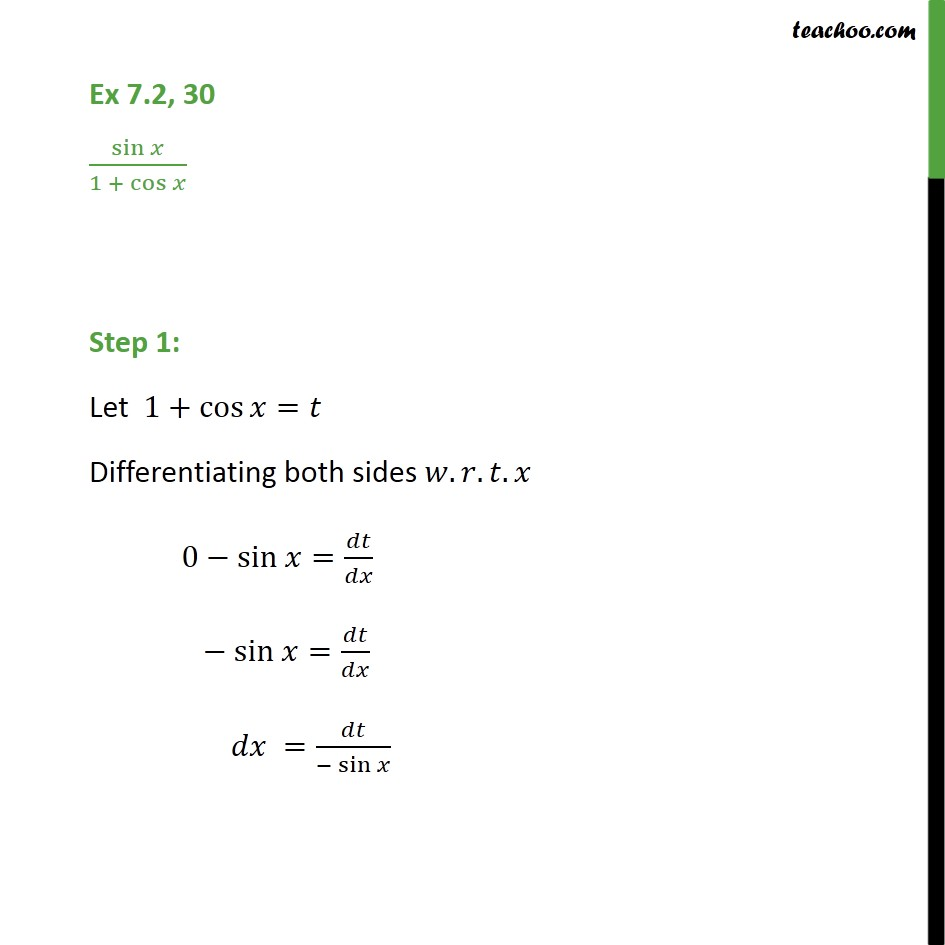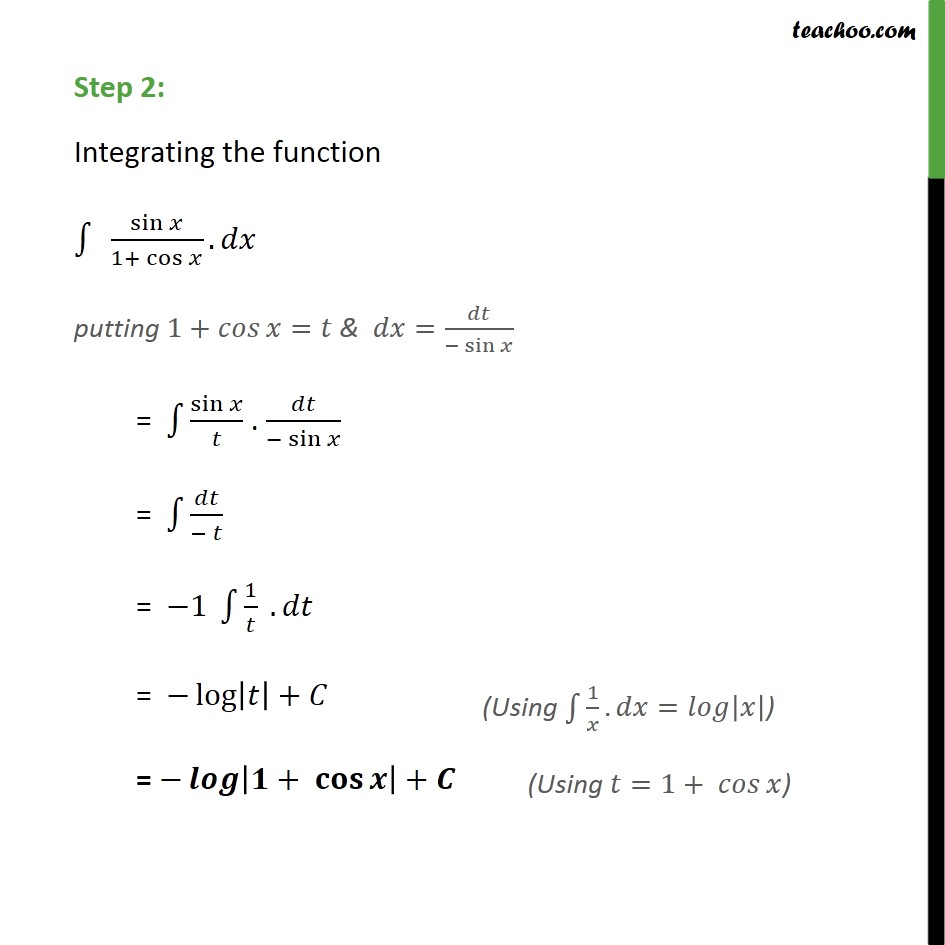1. Chapter 7 Class 12 Integrals (Term 2)
2. Concept wise
3. Integration by substitution - Trignometric - Normal

Transcript

Ex 7.2, 30 sin 1 + cos Step 1: Let 1+ cos = Differentiating both sides . . . 0 sin = sin = = sin Step 2: Integrating the function sin 1+ cos . putting 1+ = & = sin = sin . sin = = 1 1 . = log + = + +